# Tag: Elkies

Most chess programs are able to give a numerical evaluation of a position. For example, the position below is considered to be worth +8.7 with white to move, and, -0.7 with black to move (by a certain program). But, if one applies combinatorial game theory as in John Conway’s ONAG and the Berlekamp-Conway-Guy masterpiece Winning Ways for your Mathematical Plays it will turn out that the position can be proved to have an infinitesimal advantage for white…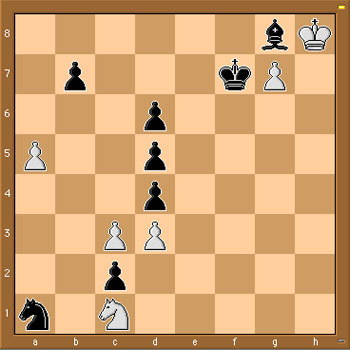So, what do we mean by this? First some basic rules of combinatorial game theory. To start, we evaluate a position without knowing which player has the move. A zero-game is by definition a position in which neither player has a good move, that is, any move by either player quickly leads to losing the game. Hence, a zero-game is a position in which the second player to move wins.

What is the chess-equivalent of a zero-position game? A position in which neither player has a good move is called a Mutual Zugzwang in chess literature. An example is given by the above position, if we restrict attention only to the 4 pieces in the upper right-hand corner and forget the rest. We don’t know who has the move, but, White cannot move at all and Black cannot move the King or Bishop without losing the Bishop and allowing White to promote the pawn and win quickly. In CGT-parlance, the upper-right position has value $\{ \emptyset | \emptyset \} = 0$ where the left options denote the White moves and the right options the Black moves.

All other values are determined by recursion. For example, consider a position in which White has just one move left before the sitution is again a Mutual Zugzwang, and, Black has no good move whatsoever. After white’s move, the position will again be a zero-position and Black has no options, so the value of this position would be denoted by $\{ 0 | \emptyset \}$ and we call the value of this position to be $+1$. Similarly, if white has no options and black has one final move to make, the position would be considered to have value $\{ \emptyset | 0 \}= -1$.

Clearly, these are just the three easiest game-values to have and the real kick comes further down the road when one can prove by recursion that some games have non-integer values (such as $\{ 0 | 1 \} = \frac{1}{2}$ for a position in which white has one move to get to a mutual zugzwang and black has a move leading to a position of value $+1$ (defined as before)), or non-number values such as $\ast = \{ 0 | 0 \}$ where both white and black’s best move is to get to a mutal zugzwang. Game-values such as $\ast$ are called fuzzy (or confused with zero) and are defined by the property that the first player to move wins.

Similarly, positive game-values are those positions where White wins, independent of who has the move and negatives are those that Black wins. There is a whole menagery of game-values and the WinningWays-booklets give an example based introduction to this fascinating theory.

Brief as this introduction was, it will allow us to determine the exact value of the position in the above diagram. We know already that we can forget about the right-hand upper corner (as this is a zero-position) and concentrate attention to the left-hand side of the board.

It is easy to see that neither Knight can move without loosing quickly, nor can the pawns on a5 and b7. That is, white has just 2 options : either c3-c4 (quickly loosing after d5xc4 2. d3xc4,d4-d3 3. Nc1xd3,Na1-b3) or, and this is the only valid option c3xd4 leading to the position on the left below. Black has only one valid move : d4xc3 leading to the position on the right below.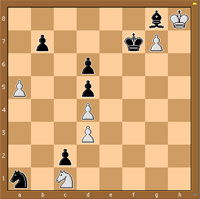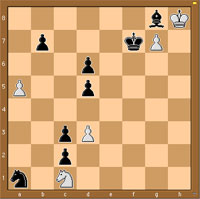Clearly, the left-diagram has value 0 as it is a mutual Zugzwang. The position on the right takes a moment’s thought : White has one move left d3-d4 leading to a 0-position, whereas black has one move d5-d4 leading to a position of value -1 (as black still has one move left d6-d5, whereas white has none). That is, the CGT-value of the right-hand position is $\{ 0 | -1 \}$ and therefore, the value of the starting position is precisely equal to

$\{ 0 | \{ 0 | -1 \} \} = +_{1}$

(called tiny-one among ONAGers)

It can be shown that $+_1$ has a positive value (that is, White wins independently of who has the first move) but smaller than any positive number-valued games!

Noam Elkies has written a beautiful paper On numbers and endgames: Combinatorial game theory in chess endgames containing many interesting examples (the example above is an adaptation of his diagram9).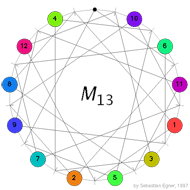Conway’s puzzle M(13) involves the 13 points and 13 lines of $\mathbb{P}^2(\mathbb{F}_3)$. On all but one point numbered counters are placed holding the numbers 1,…,12 and a move involves interchanging one counter and the ‘hole’ (the unique point having no counter) and interchanging the counters on the two other points of the line determined by the first two points. In the picture on the left, the lines are respresented by dashes around the circle in between two counters and the points lying on this line are those that connect to the dash either via a direct line or directly via the circle. In the first part we saw that the group of all reachable positions in Conway’s M(13) puzzle having the hole at the top positions contains the sporadic simple Mathieu group $M_{12}$ as a subgroup. To see the reverse inclusion we have to recall the definition of the ternary Golay code named in honour of the Swiss engineer Marcel Golay who discovered in 1949 the binary Golay code that we will encounter _later on_.

The ternary Golay code $\mathcal{C}_{12}$ is a six-dimenional subspace in $\mathbb{F}_3^{\oplus 12}$ and is spanned by its codewords of weight six (the Hamming distance of $\mathcal{C}_{12}$ whence it is a two-error correcting code). There are $264 = 2 \times 132$ weight six codewords and they can be obtained from the 132 hexads, we encountered before as the winning positions of Mathieu’s blackjack, by replacing the stars by signs + or – using the following rules. By a tet (from tetracodeword) we mean a 3×4 array having 4 +-signs indicating the row-positions of a tetracodeword. For example

$~\begin{array}{|c|ccc|} \hline & + & & \\ + & & + & \\ & & & + \\ \hline + & 0 & + & – \end{array}$ is the tet corresponding to the bottom-tetracodeword. $\begin{array}{|c|ccc|} \hline & + & & \\ & + & & \\ & + & & \\ \hline & & & \end{array}$ A col is an array having +-signs along one of the four columns. The signed hexads will now be the hexads that can be written as $\mathbb{F}_3$ vectors as (depending on the column-distributions of the stars in the hexad indicated between brackets)

$col-col~(3^20^2)\qquad \pm(col+tet)~(31^3) \qquad tet-tet~(2^30) \qquad \pm(col+col-tet)~(2^21^2)$

For example, the hexad on the right has column-distribution $2^30$ so its signed versions are of the form tet-tet. The two tetracodewords must have the same digit (-) at place four (so that they cancel and leave an empty column). It is then easy to determine these two tetracodewords giving the signed hexad (together with its negative, obtained by replacing the order of the two codewords)

$\begin{array}{|c|ccc|} \hline \ast & \ast & & \\ \ast & & \ast & \\ & \ast & \ast & \\ \hline – & + & 0 & – \end{array}$ signed as
$\begin{array}{|c|ccc|} \hline + & & & \\ & & & \\ & + & + & + \\ \hline 0 & – & – & – \end{array} – \begin{array}{|c|ccc|} \hline & + & & \\ + & & + & \\ & & & + \\ \hline + & 0 & + & – \end{array} = \begin{array}{|c|ccc|} \hline + & – & & \\ – & & – & \\ & + & + & \\ \hline – & + & 0 & – \end{array}$

and similarly for the other cases. As Conway&Sloane remark ‘This is one of many cases when the process is easier performed than described’.

We have an order two operation mapping a signed hexad to its negative and as these codewords span the Golay code, this determines an order two automorphism of $\mathcal{C}_{12}$. Further, forgetting about signs, we get the Steiner-system S(5,6,12) of hexads for which the automorphism group is $M_{12}$ hence the automorphism group op the ternary Golay code is $2.M_{12}$, the unique nonsplit central extension of $M_{12}$.

Right, but what is the connection between the Golay code and Conway’s M(13)-puzzle which is played with points and lines in the projective plane $\mathbb{P}^2(\mathbb{F}_3)$? There are 13 points $\mathcal{P}$ so let us consider a 13-dimensional vectorspace $X=\mathbb{F}_3^{\oplus 13}$ with basis $x_p~:~p \in \mathcal{P}$. That is a vector in X is of the form $\vec{v}=\sum_p v_px_p$ and consider the ‘usual’ scalar product $\vec{v}.\vec{w} = \sum_p v_pw_p$ on X. Next, we bring in the lines in $\mathbb{P}^2(\mathbb{F}_3)$.

For each of the 13 lines l consider the vector $\vec{l} = \sum_{p \in l} x_p$ with support the four points lying on l and let $\mathcal{C}$ be the subspace (code) of X spanned by the thirteen vectors $\vec{l}$. Vectors $\vec{c},\vec{d} \in \mathcal{C}$ satisfy the remarkable identity $\vec{c}.\vec{d} = (\sum_p c_p)(\sum_p d_p)$. Indeed, both sides are bilinear in $\vec{c},\vec{d}$ so it suffices to check teh identity for two line-vectors $\vec{l},\vec{m}$. The right hand side is then 4.4=16=1 mod 3 which equals the left hand side as two lines either intersect in one point or are equal (and hence have 4 points in common). The identity applied to $\vec{c}=\vec{d}$ gives us (note that the squares in $\mathbb{F}_3$ are {0,1}) information about the weight (that is, the number of non-zero digits) of codewords in $\mathcal{C}$

$wt(\vec{c})~mod(3) = \sum_p c_p^2 = (\sum_p c_p)^2 \in \{ 0,1 \}$

Let $\mathcal{C}’$ be the collection of $\vec{c} \in \mathcal{C}$ of weight zero (modulo 3) then one can verify that $\mathcal{C}’$ is the orthogonal complement of $\mathcal{C}$ with respect to the scalar product and that the dimension of $\mathcal{C}$ is seven whereas that of $\mathcal{C}’$ is six.
Now, let for a point p be $\mathcal{G}_p$ the restriction of

$\mathcal{C}_p = \{ c \in \mathcal{C}~|~c_p = – \sum_{q \in \mathcal{P}} c_q \}$

to the coordinates of $\mathcal{P} – \{ p \}$, then $\mathcal{G}_p$ is clearly a six dimensional code in a 12-dimensional space. A bit more work shows that $\mathcal{G}_p$ is a self-dual code with minimal weight greater or equal to six, whence it must be the ternary Golay code! Now we are nearly done. _Next time_ we will introduce a reversi-version of M(13) and use the above facts to deduce that the basic group of the Mathieu-groupoid indeed is the sporadic simple group $M_{12}$.

References

Robert L. Griess, “Twelve sporadic groups” chp. 7 ‘The ternary Golay code and $2.M_{12}$’

John H. Conway and N. J.A. Sloane, “Sphere packings, lattices and groups” chp 11 ‘The Golay codes and the Mathieu groups’

John H. Conway, Noam D. Elkies and Jeremy L. Martin, ‘The Mathieu group $M_{12}$ and its pseudogroup extension $M_{13}$’ arXiv:math.GR/0508630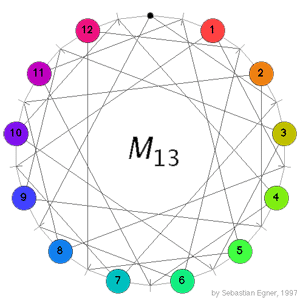Conway’s puzzle M(13) is a variation on the 15-puzzle played with the 13 points in the projective plane $\mathbb{P}^2(\mathbb{F}_3)$. The desired position is given on the left where all the counters are placed at at the points having that label (the point corresponding to the hole in the drawing has label 0). A typical move consists in choosing a line in the plane going through the point where the hole is, choose one of the three remaining points on this line and interchange the counter on it for the hole while at the same time interchanging the counters on the other two points. In the drawing on the left, lines correspond to the little-strokes on the circle and edges describe which points lie on which lines. For example, if we want to move counter 5 to the hole we notice that both of them lie on the line represented by the stroke just to the right of the hole and this line contains also the two points with counters 1 and 11, so we have to replace these two counters too in making a move. Today we will describe the groupoid corresponding to this slide-puzzle so if you want to read on, it is best to play a bit with Sebastian Egner’s M(13) Java Applet to see the puzzle in action (and to use it to verify the claims made below). Clicking on a counter performs the move taking the counter to the hole.

Recently, I’ve been playing with the idea of writing a book for the general public. Its title is still unclear to me (though an idea might be “The disposable science”, better suggestions are of course wellcome) but I’ve fixed the subtitle as “Mathematics’ puzzling fall from grace”. The book’s concept is simple : I would consider the mathematical puzzles creating an hype over the last three centuries : the 14-15 puzzle for the 19th century, Rubik’s cube for the 20th century and, of course, Sudoku for the present century.

For each puzzle, I would describe its origin, the mathematics involved and how it can be used to solve the puzzle and, finally, what the differing quality of these puzzles tells us about mathematics’ changing standing in society over the period. Needless to say, the subtitle already gives away my point of view. The final part of the book would then be more optimistic. What kind of puzzles should we promote for mathematical thinking to have a fighting chance to survive in the near future?

Here a list of pdf-files of NeverEndingBooks-posts on games, in reverse chronological order.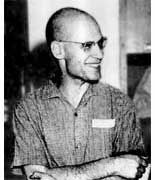The Oscar in
the category The Best Rejected Research Proposal in Mathematics
(ever)
goes to … Alexander Grothendieck
for his proposal Esquisse d’un Programme, Grothendieck\’s research program from 1983, written as
part of his application for a position at the CNRS, the French
equivalent of the NSF. An English translation is
available.

Here is one of the problems discussed :
Give TWO non-trivial elements of
$Gal(\overline{\mathbb{Q}}/\mathbb{Q})$
the _absolute_
Galois group of the algebraic closure of the rational numbers
$\overline{\mathbb{Q}}$, that is the group of all
$\mathbb{Q}$-automorphisms of $\overline{\mathbb{Q}}$. One element
most of us can give (complex-conjugation) but to find any other
element turns out to be an extremely difficult task.

To get a handle on
this problem, Grothendieck introduced his _’Dessins d’enfants’_
(Children’s drawings). Recall from last session the pictures of the
left and right handed Monsieur Mathieu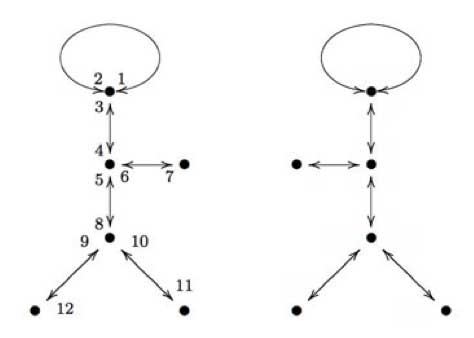The left hand side drawing was associated to a map
$\mathbb{P}^1_{\mathbb{C}} \rightarrow \mathbb{P}^1_{\mathbb{C}}$ which was
defined over the field $\mathbb{Q} \sqrt{-11}$ whereas the right side
drawing was associated to the map given when one applies to all
coefficients the unique non-trivial automorphism in the Galois group
$Gal(\mathbb{Q}\sqrt{-11}/\mathbb{Q})$ (which is
complex-conjugation). Hence, the Galois group
$Gal(\mathbb{Q}\sqrt{-11}/\mathbb{Q})$ acts _faithfully_ on the
drawings associated to maps $\mathbb{P}^1_{\mathbb{Q}\sqrt{-11}} \rightarrow \mathbb{P}^1_{\mathbb{Q}\sqrt{-11}}$ which are ramified only over
the points ${ 0,1,\infty }$.

Grothendieck’s idea was to
extend this to more general maps. Assume that a projective smooth curve
(a Riemann surface) X is defined over the algebraic numbers
$\overline{\mathbb{Q}}$ and assume that there is a map $X \rightarrow \mathbb{P}^1_{\mathbb{C}}$ ramified only over the points
${ 0,1,\infty }$, then we can repeat the procedure of last time and
draw a picture on X consisting of d edges (where d is the degree
of the map, that is the number of points lying over another point of
$\mathbb{P}^1_{\mathbb{C}}$) between white resp. black points (the
points of X lying over 1 (resp. over 0)).

Call such a drawing a
‘dessin d\’enfant’ and look at the collection of ALL dessins
d’enfants associated to ALL such maps where X runs over ALL curves
defined over $\overline{\mathbb{Q}}$. On this set, there is an action
of the absolute Galois group
$Gal(\overline{\mathbb{Q}}/\mathbb{Q})$ and if this action would be
faithful, then this would give us insight into this
group. However, at that time even the existence of a map $X \rightarrow \mathbb{P}^1$ ramified in the three points ${ 0,1,\infty }$
seemed troublesome to prove, as Grothendieck recalls in his proposal

In more erudite terms, could it be true that
every projective non-singular algebraic curve defined over a number
field occurs as a possible ‚ modular curve‚ parametrising
elliptic curves equipped with a suitable rigidification? Such a
supposition seemed so crazy that I was almost embarrassed to submit
it to the competent people in the domain. Deligne when I consulted
him found it crazy indeed, but didn’t have any counterexample up
his sleeve. Less than a year later, at the International Congress in
Helsinki, the Soviet mathematician Bielyi announced exactly that result,
with a proof of disconcerting simplicity which fit into two little
pages of a letter of Deligne ‚ never, without a doubt, was such a
deep and disconcerting result proved in so few lines!

In
the form in which Bielyi states it, his result essentially says that
every algebraic curve defined over a number field can be obtained as
a covering of the projective line ramified only over the points 0,
1 and infinity. This result seems to have remained more or less
unobserved. Yet, it appears to me to have considerable importance. To
me, its essential message is that there is a profound identity
between the combinatorics of finite maps on the one hand, and the
geometry of algebraic curves defined over number fields on the
other. This deep result, together with the algebraic- geometric
interpretation of maps, opens the door onto a new, unexplored world within reach of all, who pass by without seeing it.

Belyi’s proof is indeed relatively easy
(full details can be found in the paper Dessins d’enfants on the
Riemann sphere
by Leila
Schneps). Roughly it goes as follows : as both X and the map are
defined over $\overline{\mathbb{Q}}$ the map is only ramified over
(finitely many) $\overline{\mathbb{Q}}$-points. Let S be the finite
set of all Galois-conjugates of these points and consider the polynomial

$f_0(z_0) = \prod_{s \in S} (z_0 -s) \in \mathbb{Q}[z_0]$

Now, do a
resultant trick. Consider the
polynomial $f_1(z_1) = Res_{z_0}(\frac{d f_0}{d z_0},f_0(z_0)-z_1)$ then the roots of $f_1(z_1)$ are exactly the
finite critical values of $f_0$, $f_1$ is again defined over
$\mathbb{Q}$ and has lower degree (in $z_1$) than $f_0$ in $z_1$.
Continue this trick a finite number of times untill you have constructed
a polynomial $f_n(z_n) \in \mathbb{Q}[z_n]$ of degree zero.

Composing
the original map with the maps $f_j$ in succession yields that all
ramified points of this composition are
$\mathbb{Q}$-points! Now, we only have to limit the number of
these ramified $\mathbb{Q}$-points (let us call this set T) to three.

Take any three elements of T, then there always exist integers $m,n \in \mathbb{Z}$ such that the three points go under a linear
fractional transformation (a Moebius-function associated to a matrix in
$PGL_2(\mathbb{Q})$) to ${ 0,\frac{m}{m+n},1 }$. Under the
transformation $z \rightarrow \frac{(m+n)^{m+n}}{m^m n^n}z^m(1-z)^n$ the points 0 and 1 go to 0 and
$\frac{m}{m+n}$ goes to 1 whence the ramified points of the
composition are one less in number than T. Continuing in this way we
can get the set of ramified $\mathbb{Q}$-points of a composition at
most having three elements and then a final Moebius transformation gets
them to ${ 0,1,\infty }$, done!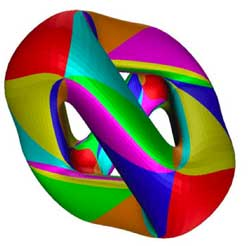As a tribute for this clever
argument, maps $X \rightarrow \mathbb{P}^1$ ramified only in 0,1 and
$\infty$ are now called Belyi morphisms. Here is an example of
a Belyi-morphism (and the corresponding dessin d’enfants) associated to
one of the most famous higher genus curves around : the Klein
quartic
(if you haven’t done
so yet, take your time to go through this marvelous pre-blog post by
John Baez).

One can define the Klein quartic as the plane projective
curve K with defining equation in
$\mathbb{P}^2_{\\mathbb{C}}$ given by $X^3Y+Y^3Z+Z^3X = 0$ K has
a large group of automorphism, namely the simple group of order
168 $G = PSL_2(\mathbb{F}_7) = SL_3(\mathbb{F}_2)$ It is a classical fact (see for example
the excellent paper by Noam Elkies The Klein quartic in number theory) that the quotient map $K \rightarrow K/G = \mathbb{P}^1_{\mathbb{C}}$ is ramified only in the points
0,1728 and $\infty$ and the number of points of K lying over them
are resp. 56, 84 and 24. Now, compose this map with the Moebius
transormation taking ${ 0,1728,\infty } \rightarrow { 0,1,\infty }$
then the resulting map is a Belyi-map for the Klein quartic. A
topological construction of the Klein quartic is fitting 24 heptagons
together so that three meet in each vertex, see below for the gluing
data-picture in the hyperbolic plane : the different heptagons are given
a number but they appear several times telling how they must fit
together)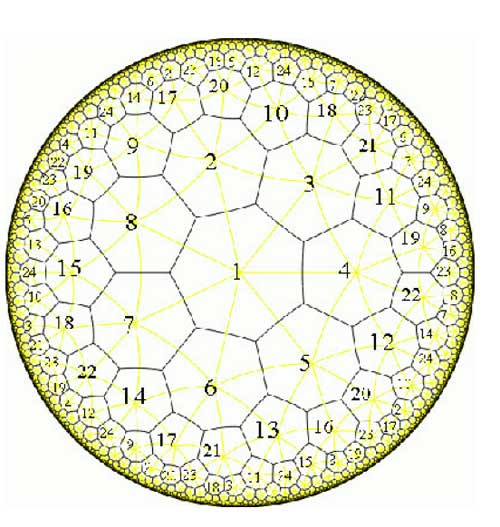The resulting figure has exactly $\frac{7 \times 24}{2} = 84$ edges and the 84 points of K lying over 1 (the white points in
the dessin) correspond to the midpoints of the edges. There are exactly
$\frac{7 \times 24}{3}=56$ vertices corresponding to the 56 points
lying over 0 (the black points in the dessin). Hence, the dessin
d\’enfant associated to the Klein quartic is the figure traced out by
the edges on K. Giving each of the 168 half-edges a
different number one assigns to the white points a permutation of order
two and to the three-valent black-points a permutation of order three,
whence to the Belyi map of the Klein quartic corresponds a
168-dimensional permutation representation of $SL_2(\mathbb{Z})$,
which is not so surprising as the group of automorphisms is
$PSL_2(\mathbb{F}_7)$ and the permutation representation is just the
regular representation of this group.

Next time we will see how
one can always associate to a curve defined over
$\overline{\mathbb{Q}}$ a permutation representation (via the Belyi
map and its dessin) of one of the congruence subgroups $\Gamma(2)$ or
$\Gamma_0(2)$ or of $SL_2(\mathbb{Z})$ itself.

2005
was the year that the DaVinci code craze hit Belgium. (I started reading Dan Brown’s
Digital Fortress and Angels and Demons a year
before on the way back from a Warwick conference and when I read DVC a
few months later it was an anti-climax…). Anyway, what better way
to end 2005 than with a fitting chess problem, composed by Noam Elkies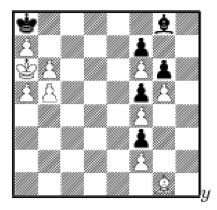The problem is to give an infinite sequence
of numbers, the n-th term of the sequence being the number of ways White
can force checkmate in exactly n moves. With the DVC-hint given, clearly
only one series can be the solution… To prove it, note that
White’s only non-checkmating moves are with the Bishop traveling
along the path (g1,h2,g3,h4) and use symmetry to prove that the number
of paths of length exactly k starting from h2 is the same as those
starting from g3…

If that one was too easy for you,
consider the same problem for the position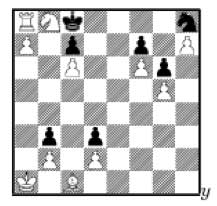Here the solution are the 2-powers of those
of the first problem. The proof essentially is that White has now two
ways to deliver checkmate : Na6 and Nd7… For the solutions and
more interesting chess-problems consult Noam Elkies’ excellent
paper New directions in
enumerative chess problems
. Remains the problem which sequences can
arise on an $N \\times N$ board with an infinite supply of chess
pieces!

Noam Elkies is one of
those persons I seem to bump into (figuratively speaking) wherever my
interests take me. At the moment I’m reading (long overdue, I
know, I know) the excellent book Notes on
Fermat’s Last Theorem
by Alf Van der
Poorten
. On page 48, Elkies figures as an innocent bystander in the
1994 April fools joke e-perpetrated by
Henri Darmon
in the midst of all confusion about ‘the
gap’ in Wiles’ proof.

There has
been a really amazing development today on Fermat’s Last Theorem.
Noam Elkies has announced a counterexample, so that FLT is not true
Fermat that he constructs involves an incredibly large prime exponent
(larger than $10^{20}$), but it is constructive. The main idea seems to
be a kind of Heegner-point construction, combined with a really
ingenious descent for passing from the modular curves to the Fermat
curve. The really difficult part of the argument seems to be to show
that the field of definition of the solution (which, a priori, is some
ring class field of an imaginary quadratic field) actually descends to
$\\mathbb{Q}$. I wasn’t able to get all the details, which were
quite intricate…

Elkies is also an
excellent composer of chess problems. The next two problems he composed
as New Year’s greetings. The problem is : “How many shortest
sequences exists (with only white playing) to reach the given
position?”

$\\begin{position} \\White(Kb5,Qd1,Rb1,Rh1,Nc3,Ne5,Bc1,Bf1,a2,b2,c4,d2,e2,f3,g3,h2) \\end{position}{\\font\\mathbb{C}hess=chess10 \\showboard }xc$

Here’s Elkies’ solution
:

There are 2004 sequences of the minimal length 12.
Each consists of the sin- gle move g3, the 3-move sequence
c4,Nc3,Rb1, and one of the three 8-move sequences
Nf3,Ne5,f3,Kf2,Ke3,Kd3(d4),Kc4(c5),Kb5. The move g3 may be played at
any point, and so contributes a factor of 12. If the King goes
through c5 then the 3- and 8-move sequences are independent, and can
be played in $\\binom{11}{3}$ orders. If the King goes through c4 then
the entire 8-move sequence must be played before the 3-move sequence
begins, so there are only two possibilities, depending on the choice
of Kd3 or Kd4. Hence the total count is $12(\\binom{11}{3}+2)=2004$ as
claimed.

A year later he composed the
problem

$\\begin{position} \\White(Kh3,Qe4,Rc2,Rh1,Na4,Ng1,Bc1,Bf1,a2,b2,c3,d3,e2,f4,g2,h2) \\end{position}{\\font\\mathbb{C}hess=chess10 \\showboard }xd$

of which Elkies’ solution is
:

There are 2005 sequences of the minimal length 14.
This uses the happy coincidence $\\binom{14}{4}=1001$. Here White
plays the 4-move sequence f4,Kf2,Kg3,Kh3 and one of the five
sequences Nc3,Na4,c3,Qc2,Qe4,d3,Bd2(e3,f4,g5,h6),Rc1,Rc2,Bc1 of
length 10. If the Bishop goes to d2 or e3, the sequences are
independent, and can be played in $\\binom{14}{4}$ orders. Otherwise
the Bishop must return to c1 before White plays f4, so the entire
10-move sequence must be played before the 4-move sequence begins. Hence
the total count is $2 \\binom{14}{4}+3 = 2005$.

With just a few weeks remaining, anyone in for
a 2006 puzzle?

Klein’s
quartic $X$is the smooth plane projective curve defined by
$x^3y+y^3z+z^3x=0$ and is one of the most remarkable mathematical
objects around. For example, it is a Hurwitz curve meaning that the
finite group of symmetries (when the genus is at least two this group
can have at most $84(g-1)$ elements) is as large as possible, which in
the case of the quartic is $168$ and the group itself is the unique
simple group of that order, $G = PSL_2(\mathbb{F}_7)$ also known as
Klein\’s group. John Baez has written a [beautiful page](http://math.ucr.edu/home/baez/klein.html) on the Klein quartic and
its symmetries. Another useful source of information is a paper by Noam
Elkies [The Klein quartic in number theory](www.msri.org/publications/books/Book35/files/elkies.pd).
The quotient map $X \rightarrow X/G \simeq \mathbb{P}^1$ has three
branch points of orders $2,3,7$ in the points on $\mathbb{P}^1$ with
coordinates $1728,0,\infty$. These points correspond to the three
non-free $G$-orbits consisting resp. of $84,56$ and $24$ points.
Now, remove from $X$ a couple of $G$-orbits to obtain an affine open
subset $Y$ such that $G$ acts on its cordinate ring $\mathbb{C}[Y]$ and
form the Klein stack (or hereditary order) $\mathbb{C}[Y] \bigstar G$,
the skew group algebra. In case the open subset $Y$ contains all
non-free orbits, the [one quiver](www.matrix.ua.ac.be/master/coursenotes/onequiver.pdf) of this
qurve has the following shape $\xymatrix{\vtx{} \ar@/^/[dd] \\ \\ \vtx{} \ar@/^/[uu]}$ $\xymatrix{& \vtx{} \ar[ddl] & \\ & & \\ \vtx{} \ar[rr] & & \vtx{} \ar[uul]}$ $\xymatrix{& & \vtx{} \ar[dll] & & \\ \vtx{} \ar[d] & & & & \vtx{} \ar[ull] \\ \vtx{} \ar[dr] & & & & \vtx{} \ar[u] \\ & \vtx{} \ar[rr] & & \vtx{} \ar[ur] &}$ Here, the three components correspond to the three
non-free orbits and the vertices correspond to the isoclasses of simple
$\mathbb{C}[Y] \bigstar G$ of dimension smaller than $168$. There are
two such of dimension $84$, three of dimension $56$ and seven of
dimension $24$ which I gave the non-imaginative names \’twins\’,
\’trinity\’ and \’the dwarfs\’. As we want to spice up later this
Klein stack to a larger group, we need to know the structure of these
exceptional simples as $G$-representations. Surely, someone must have
written a paper on the general problem of finding the $G$-structure of
simples of skew-group algebras $A \bigstar G$, so if you know a
reference please let me know. I used an old paper by Idun Reiten and
Christine Riedtmann to do this case (which is easier as the stabilizer
subgroups are cyclic and hence the induced representations of their
one-dimensionals correspond to the exceptional simples).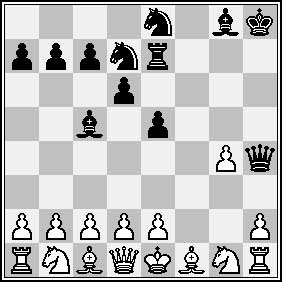Noam D. Elkies is a
Harvard mathematician whose main research interests have to do with
lattices and elliptic curves. He is also a very talented composer of
chess problems. The problem to teh left is a proof game
in 14 moves. That is, find the UNIQUE legal chess game leading to the
given situation after the 14th move by black. Elkies has also written a
beautiful paper On Numbers
and Endgames
applying combinatorial game theory (a la Winning
Ways!) to chess-endgames (mutual Zugzwang positions correspond to zero
positions) and a follow-up article Higher Nimbers in pawn
endgames on large chessboards
. Together with Richard Stanley he wrote a
paper for the Mathematical Intelligencer called The Mathematical
Knight
which is stuffed with chess problems! But perhaps most
surprising is that he managed to run his own course on Chess and
Mathematics
!